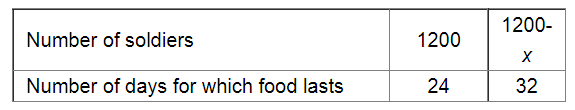# 1200 soldiers in a fort had enough food for 28 days.`
Question:

1200 soldiers in a fort had enough food for 28 days. After 4 days, some soldiers were transferred to another fort and thus the food lasted now for 32 more days. How many soldiers left the fort?

Solution:

It is given that after 4 days, out of 28 days, the fort had enough food for 1200 soldiers for $(28-4=24)$ days.

Let $x$ be the number of soldiers who left the fort.Since the number of soldiers and the number of days for which the food lasts are in inverse variation, we have :

$1200 \times 24=(1200-x) \times 32$

$\Rightarrow \frac{1200 \times 24}{32}=1200-x$

$\Rightarrow 900=1200-x$

$\Rightarrow x=1200-900$

$=300$

Thus, 300 soldiers left the fort.